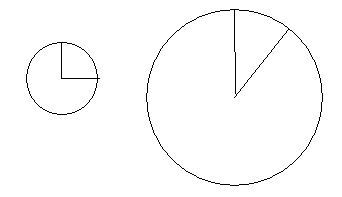Question from Dani: How do I draw the arc (calculate the included angle) when I know only the arc length? Hi Dani. You can't do it. If you don't know the radius of the circle (assuming you mean a circular arc), then there are lots of arcs with the same length - for different sizes of circles. And each radius means a different included angle. Here's a diagram to demonstrate:Stephen La Rocque. >